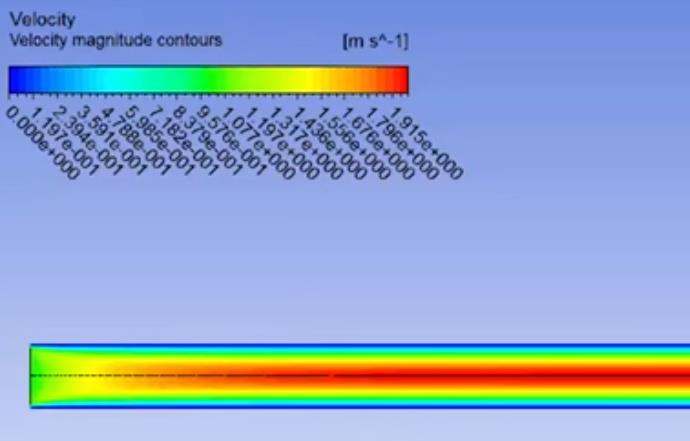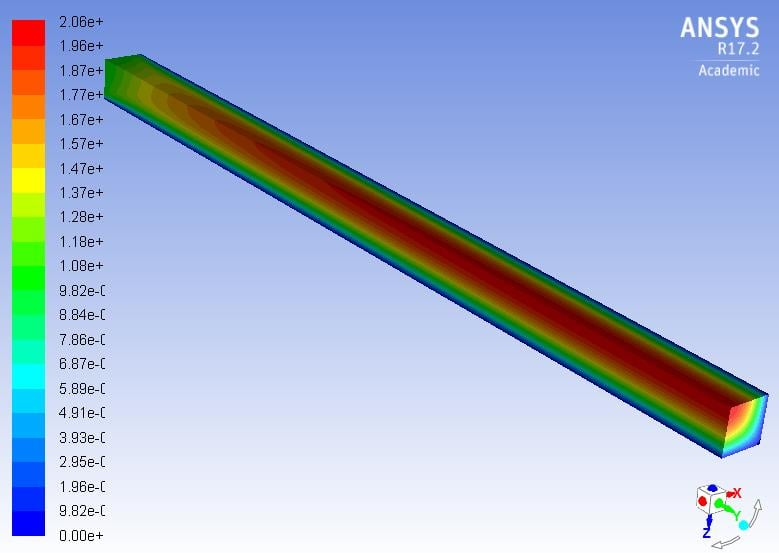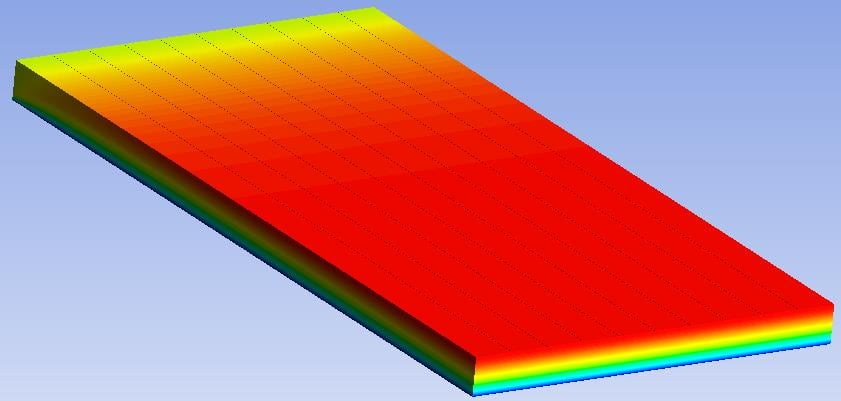# 2D space for planar and axisymmetric computation domainhi all,

recently, I've been confused about an example in a fluent tutorial. the above picture shows the real geometry of the computation domain. in specific, it's a cylindrical pipe with porous filtering media inside.

the computation domain is two-dimensional. after imported into fluent, it is selected to be planer in 2D space panel instead of axisymmetric in the tutorial. I am wondering does planar mean infinitely big plane? as far as I am concerned, cylindrical pipe is definitely not infinitely big planes. or am I misunderstanding the meaning of planar?

can anybody help me to understand it ?

thanks very much

weiqiang

• Hi weiqiang,

Axisymmetric Fluent models must have the rotational axis of symmetry along the global X-axis, and the radial slice geometry drawn in the positive Y space. The equations compute the 3D solution by using the 2D radial slice. Therefore a rectangle sitting on the X axis represents a cylinder of fluid domain. To convert the diagram of a 3D object into an axisymmetric model, the elbow and and pipe from the side must be excluded and the inlet and outlet be created on the axis.

Planar Fluent models can be drawn anywhere in the X-Y plane.  They represent an infinitely deep (Z-axis) fluid domain.

Hope this helps,
Peter

• edited December 2018

hi Peter,

thanks very much for your reply. yes, I can understand meaning of planar or axisymmetric. however, the geometry in the picture is obviously not infinitely deep along Z-axis. Instead, it's a cylindrical structure part by part. how can the author select planar in 2D space panel?

Weiqiang

• Hi Weiqiang,

I think it is more reasonable to build an axisymmetric model of that drawing. I don't see the benefit of a planar 2D model.

Regards,
Peter

• edited December 2018

yes maybe I don't have to believe a not very official tutorial

thanks

weiqiang

• edited December 2018
Adding on to the discussion, for 2D Planar cases, the depth is usually set to unit length. You can set the depth by setting it in the “Reference Values” panel.

As Peter mentioned, it’s the inlet and outlet positioning that is probably why 2D axisymmetric was not used in tutorial. If your inlet Reynolds number is low, you can neglect the inlet and outlet and model it as an axisymmetric case.
• edited December 2018

hi Rahul,

so if the depth is usually set to unit length, the real 3D geometry is cubic ? if it's a like a square channel, how can a plane represent the whole computation domain because of the viscous effect of wall?

as far as I am concerned, the flow field in a square channel can never be simplified into 2D planar. Because you can not find a plane to represent the whole computation domain, am I right?

It really confuses me a lot.

thanks a lot

weiqiang

• Hi Weiqiang,

A unit depth is just so you can calculate quantities "per unit depth" but it is not a 2D representation of a 3D cubic volume as there are no walls in the z plane like there would be on a real 3D duct and so there is no variation in quantities in the z direction.

This drawing just does not lend itself to an accurate representation in 2D.  The central portion could be considered axisymmetric, but the inlet conditions from the bend in the pipe would make the 3D flow quite different than what you can define in an axisymmetric inlet, so that becomes a source of error between the axisymmetric model and the true 3D flow.

Regards,
Peter

• edited December 2018

hi Peter,

so according to the definition of planar in fluent, a square straight channel can never be represent by a 2D planar. Am I understanding right?

weiqiang.

• You know a 3D object called a pipe. If we draw a rectangle from the centerline to the pipe wall, the height of the rectangle is R, the radius of the pipe, and the length is L. If we revolve that rectangle about the centerline, we can reconstruct the 3D volume of fluid in the pipe. The rectangle drawn represents the fluid domain for a 2D Axisymmetric model of the 3D volume. There is no “depth” dimension to a pipe, because it is revolved around the centerline, so the wall is always a distance R from the centerline, and the forces generated in the 2D Axisymmetric model are for the wetted surface area of the inside wall of the pipe. In post processing, we can mirror the results from the +Y side of the X-axis to the -Y side and make the image shown below.We can imagine a 3D object known as a duct. A square duct has parallel walls separated by a distance of S. The fluid volume has depth S, height S, and length L. The fluid flow in this duct cannot be reduced to a 2D flow simulation. A 3D simulation is required to calculate the flow in a duct. However, we can use symmetry to reduce the problem size. A quarter symmetry 3D model has a rectangular solid with sides of dimension S/2 and length L. A 3D model of a quarter symmetry model has two faces of the solid as wall BC, two faces of the solid as symmetry BC, and an inlet and outlet face. The result is shown below.We can imagine a pair of parallel walls separated by a distance of S with a length L. Let’s call this a channel. If the parallel walls of the channel have a depth dimension D >> S, then the flow of a fluid between the walls can be approximated in a 2D model. We can use symmetry and draw just half the channel by drawing a rectangle of height S/2 and length L. The “depth” dimension in a 2D model is considered infinite. However, for the purpose of calculating forces, a unit depth is used. This is the Planar 2D model. I built a half symmetry 3D model of this channel by constructing a rectangular solid with sides of dimension S/2 and length L. A 3D model of a half symmetry model has one face of the solid as a wall BC and three faces of the solid as symmetry BCs. The flow solution is shown below, where I used the Default Transform in CFD-Post to make 10 copies of the result to better illustrate the channel nature of the problem. Note that this 3D model has the same result as the 2D Planar model.Hope this helps to clarify the differences.

Regards,
Peter

• edited December 2018

Hi Peter,

I really appreciate your super clear illustration!! Actually, my confusion came from a paper review when I tried to reproduce the simulation results with fluent.

The object of the simulation is called diesel particulate filter, namely DPF. you might know this device. it's a particle filter of diesel engine. below is the real picture of this device.the square straight channels of DPF are open and plugged alternatively. The exhaust gas of diesel engine flows into the filter from open channels and is forced through the porous wall. Ultimately, exhaust flows out of the filter from the other end of the filter and the soot particles are left in the porous wall. The picture below shows the working principle of DPF.After the mass of soot deposited in the filter reach some predefined limitation, the soot has to be burned out which is called regeneration. I am trying to simulate this burning process in DPF with fluent. In conclude, it's a simulation case related to flow field, porous media combustion. Ok , above is the background.

I found a paper published on Chemical Engineer Science which took a pair of the channels as 2D computation domain. Below is the computation domain in this paper.Except inlet, outlet and interface of the porous media, all other boundary conditions are WALL in the paper. There are two aspects that confuse me very much.

1. Since it's a square straight channel, then it can not be simplified into 2D model like you said, right?

2. In a single channel of the DPF, all four walls are porous media, which makes all of the wall deposited with soot cake. Then why is the lower wall has porous soot cake on it and the upper wall is set to WALL boundary condition?

I sent an email to the author to express my confusion but did not get any reply.

have you ever met cases like this?

thanks very much!

weiqiang

• Hi Weiqiang,

I'm learning CFD (slowly) and haven't built a lot of models yet, but will share my opinion. Maybe others will reply with their comments.

The DPF has an inlet duct with four porous walls where exhaust gases can flow to the adjacent four outlet ducts. You could certainly build a 3D model of this. I think the author is "unwrapping" the four porous walls of one inlet duct, and putting the unwrapped porous walls flat on the X-Z plane, putting the unfiltered air on the +Y side of the porous wall and the filtered air of the four adjacent ducts on the -Y side of the porous wall, thereby creating a 2D planar model of the complex 3D device. If the depth of the 2D planar model is 4*1.4 mm, assuming the duct width is 1.4 mm, then that multiplied by the length of 30 mm represents the total filter area for that inlet duct.

The 2D planar model shown in the paper illustration shows only one duct volume of flow on the inlet side, and one duct volume on the outlet side. The 3D geometry shows that each inlet duct can flow into four outlet ducts, but also that each outlet duct is fed by four inlet ducts. So the aggregate flow volume in the 2D planar model and the total filter area are correct in a much simpler model than the 3D model. I expect the 2D planar model could predict with some accuracy the pressure drop across the filter and the change in pressure as the filter clogs.

Regards,
Peter

• edited December 2018

hi Peter,

yes, it makes sense. I have to admit you are smarter than me. I have another question regarding to this problem. If the exhaust velocity in front of a real DPF channel is 4m/s, than the inlet velocity of the 2D planar model has to be 1m/s to match the whole volume flow rate, right?

By the way, do you have some tutorials or guiding books which involve the porous media combustion simulation with fluent, in my case, soot cake combustion to recommend. I went through the official tutorial of ANSYS and some other published tutorials but only found the flow field simulation through porous media.

I really really appreciate your help!!!

Weiqiang.

• Hi Weiqiang,

If the average inlet velocity for the pipe area is 4 m/s, then an individual duct would be 8 m/s because only half the pipe area is inlet ducts, the outlet ducts being blocked at the inlet side.

But the inlet flow to an individual inlet duct is going to equal the outlet flow of an individual outlet duct, it's just that 1/4 of the flow out is coming from 4 different inlet ducts.

Sorry, I don't have any tutorials on porous media or combustion. I've never looked at that before. I see you have a new discussion. Keep asking!  If you are done with this discussion, you can mark it as Is Solution to close it out.

Good luck,
Peter

• edited January 2019

Hi Peter,

I mean if the inlet velocity of an individual duct is 4m/s, then in the 2D planar simulation model, the inlet velocity has to be set to 1m/s to match the whole volume flow rate. Am I right? Because in a 2D planar model, the volume of flow goes through only 1 ‘unwrapped’ wall.

Yes, I have a new discussion about porous media combustion. Thanks very much for your help. I think I am very very very close to Is Solution.

weiqiang